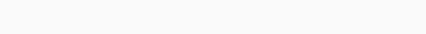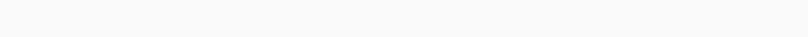# itertools.cycle() in Python

Hello Coder, this tutorial deals with a program to demonstrate the usage of cycle() method from itertools package. Learn itertools.cycle() in Python with examples.
Let’s start.

itertools: This is a package of various methods that are used to iterate with fast and efficient manner.

Mainly, iterators are three types:

• Infinite iterators:  The infinite iterators produce the infinite number of sequences.
• Short sequence iterators: This type of iterators produce sequences until a certain condition specified by the user.
• Combinators generators: This types of iterators used to produce combinations according to the input given (or)specified by the user.

### Importing itertools module:

`import itertools`

itertools.cycle(): This method prints all the values that are given as an argument to this method. And again it starts from the beginning when it reaches the end. To terminate this we need to keep a termination condition.

### Syntax of itertools.cycle():

`itertools.cycle(iterable)`

### Example of itertools.cycle() in Python

```import itertools
x=itertools.cycle([1,2,3])
for i in x:
print(i)```

```1
2
3
1
2
3
1
2
3
.
.
.
.```

#### Let us pass a string as an argument and see the example:

```from itertools import cycle
c=0
for i in cycle('Codespeedy'):
print(i,end='-')
c+=1
if(c>15):
break
```

Output:#### Let us pass a list as an argument and see the example:

```from itertools import cycle
c=0
l=['code','speedy']
for i in cycle(l):
print(i,end='-')
c+=1
if(c>10):
break
```

Output:For any more queries please comment below.

Also read: How to use Python iter() function

itertools.combinations() in Python